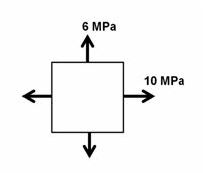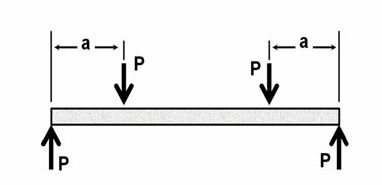Mechanical Engineering Written Exam Questions (PGCL-2010)

Company- Paschimanchal Gas Company Limited (PGCL)
Post- Assistant Engineer-Mechanical
Year- 2010
➦ Define isotropic materials and homogeneous materials, give an example of each.
➦ Define Boundary layer. Draw a sketch showing different boundary-layer flow regimes on a flat plate.
➦ Find the maximum torsional shear stress in a solid circular shaft of  diameter 10 mm, when the applied torque is 30N.m.
➦ Draw TOP and LEFT SIDE VIEW of the object shown.➦ Water flows at 8 kg/s through a diffuser with A1=7×10-4 m2 and A2=4×10-3 m2. Determine the increase in static pressure between section 1 and section 2. Assume frictionless flow and water density 1000 kg/m3➦ An elastic bar of cross section A and length L is held fixed at both ends. The bar has modulus of elasticity E and coefficient of thermal expansion α. Derive the expression for axial force due to temperature increase of ΔT. (Assume no buckling of the bar).➦ For the plane stress shown in the stress element, draw the Mohr’s circle to find the maximum in-plane shearing stress and corresponding normal stress.➦ Construct shear and bending-moment diagrams showing peak values for the simply supported beam loaded with the forces shown in figure.➦ Draw the open cycle gas turbine in simple block diagram form and show the corresponding processes on a T-S plane.
➦ What is cavitation? List any two problems caused by cavitation in turbines.
➦ Compute the pressure drop when water flows at 3 liter/s through a steel pipe with diameter 50 mm and 40 m length. Assume, density of water 1000 kg/m3 and friction factor f=0.02.
➦ A composite wall of two 0.5 m thick layers has a temperature 150oC on one face and 10oC on the other face. Thermal conductivity of layer-1 is  k1=0.05 W/m-K and layer-2 is k2=0.5 W/m-K. Consider face area A1=A2=2 m2. What is the combined thermal resistance of the wall? Find the heat transfer per unit surface area.